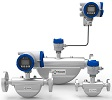Polyquip Engineering Pte Ltd |  6753 7997  | 6753 5365  | sales@polyquip.com.sg

# CORIOLIS MASS FLOWMETERA mass flow meter, also known as an inertial flow meter is a device that measures mass flow rate of a fluid traveling through a tube. The mass flow rate is the mass of the fluid traveling past a fixed point per unit time.
The mass flow meter does not measure the volume per unit time (e.g., cubic meters per second) passing through the device; it measures the mass per unit time (e.g., kilograms per second) flowing through the device. Volumetric flow rate is the mass flow rate divided by the fluid density. If the density is constant, then the relationship is simple. If the fluid has varying density, then the relationship is not simple. The density of the fluid may change with temperature, pressure, or composition, for example. The fluid may also be a combination of phases such as a fluid with entrained bubbles. Actual density can be determined due to dependency of sound velocity on the controlled liquid concentration.
Port Size: 1/16 inch to 12 inch
Flow Range: 0.0015 kg/min to 30,000 kg/min

send enquiry